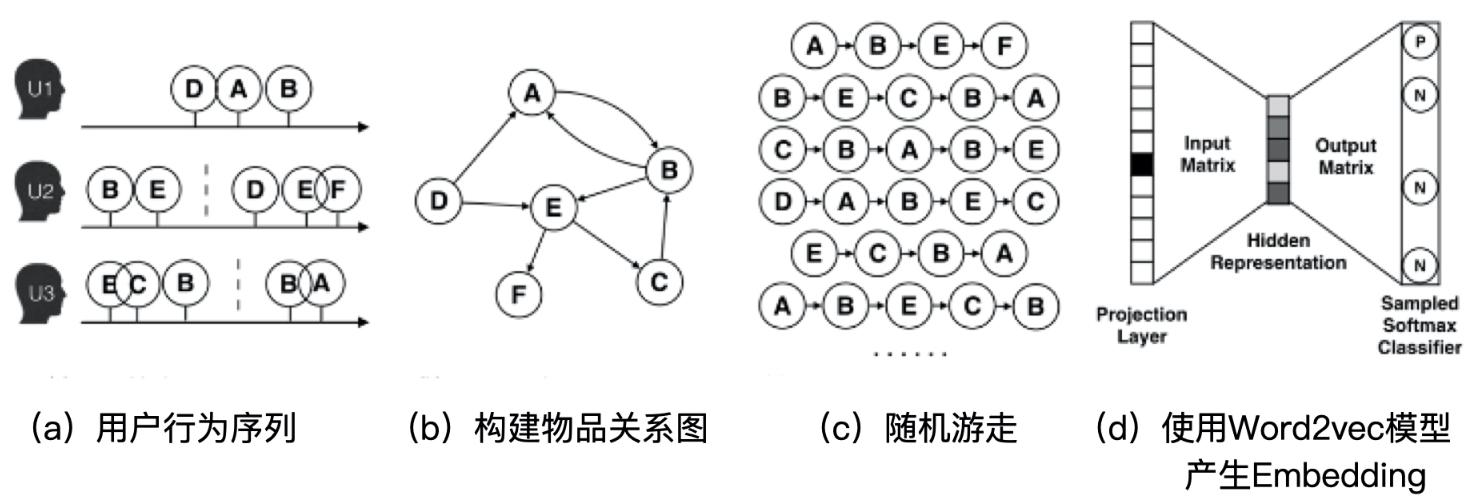V1

2023/05/04阅读：12主题：默认主题

# 推荐系统之召回

### 前言

• 基于内容的召回

• 根据item之间的相似性原则
• 召回用户喜欢的item的相似item
• 协同过滤召回

• 根据用户或物品之间的相似性原则
• 用户A与用户B相似，其中用户A喜欢item1，但是用户B并没有说明是否喜欢该item，就可以将item1推荐给用户B
• 物品同理
• 模型召回

• 矩阵分解召回
• FM模型召回
• 图网络召回
• 其他深度学习模型召回

### 内容

#### 1、基于内容的召回

• 适用于用户的初始阶段。此时用户的历史数据较少，可以通过内容召回来保证召回的数量
• 缓解内容的冷启动问题。主要指给与新内容曝光度
• 有利于捕获用户的兴趣

• 内容的特征需要人工设计，所以设计人对领域的了解程度会极大的影响结果
• 缺少多样性，也就是仅能了解用户的兴趣，无法扩展用户的兴趣

#### 2、基于协同过滤的召回

• 根据用户历史行为创建共现矩阵
• 根据共现矩阵查找相似用户
• 将相似用户喜欢的物品推荐给目标用户（即召回）

NeuralCF使用深度学习对协同过滤进行了改进

 论文：Neural Collaborative Filtering 链接：https://arxiv.org/pdf/1708.05031v2.pdf 源码：https://github.com/hexiangnan/neural_collaborative_filtering

# neural cf model arch two. only embedding in each tower, then MLP as the interaction layersdef neural_cf_model_1(feature_inputs, item_feature_columns, user_feature_columns, hidden_units):    # 物品侧特征层    item_tower = tf.keras.layers.DenseFeatures(item_feature_columns)(feature_inputs)    # 用户侧特征层    user_tower = tf.keras.layers.DenseFeatures(user_feature_columns)(feature_inputs)    # 连接层及后续多层神经网络    interact_layer = tf.keras.layers.concatenate([item_tower, user_tower])    for num_nodes in hidden_units:        interact_layer = tf.keras.layers.Dense(num_nodes, activation='relu')(interact_layer)    # sigmoid单神经元输出层    output_layer = tf.keras.layers.Dense(1, activation='sigmoid')(interact_layer)    # 定义keras模型    neural_cf_model = tf.keras.Model(feature_inputs, output_layer)    return neural_cf_model

#### 3、基于模型的召回

##### 3.1 矩阵分解

// 建立矩阵分解模型val als = new ALS()  .setMaxIter(5)  .setRegParam(0.01)  .setUserCol("userIdInt")  .setItemCol("movieIdInt")  .setRatingCol("ratingFloat")//训练模型val model = als.fit(training)//得到物品向量和用户向量model.itemFactors.show(10, truncate = false)model.userFactors.show(10, truncate = false
##### 3.2 FM模型
FM(因式分解机)在2010年由Rendle提出，核心在于通过特征组合，减少人工参与，其优点如下：
• 实现特征之间的交互
• 能处理数据高度稀疏的场景。通过对参数矩阵进行矩阵分解实现降维
• 具有线性计算复杂度
• 能够在任意的实数特征向量中生效

FM的计算公式为：

=

=

=

=

##### 3.3 图结构
###### 3.3.1 DeepWalk

2014年，美国石溪大学研究者提出随机游走。本质上就是在图结构上进行随机游走，生成item序列，这些序列将作为训练数据输入skip-gram模型，然后得到对应的embedding表示，如下图所示：生成的图结构是一种有向图，其流程如下：

• 以用户的行为序列，如购买物品序列、观看视频序列等，来构建物品关系图，如果后续产生多条相同的有向边则其权重被加强
• 随机确定起始点，使用随机游走的方式生成物品序列。其中，随机游走的长度、次数都属于超参数
• 将随机游走生成的序列输入模型生成embedding表示

###### 3.3.2 Node2vec

2016年，斯坦福大学研究员提出Node2vec。通过调整随机游走跳转概率的计算方式，让图embedding在网络的同质性和结构性中权衡。其中同质性指距离详尽的节点的embedding应该尽量近似，结构性指结构上相似的节点的embedding尽量接近。

###### 3.3.3 EGES（阿里巴巴）
 论文：Billion-scale Commodity Embedding for E-commerce Recommendation in Alibaba 链接：https://arxiv.org/abs/1803.02349

2018年，阿里巴巴提出EGES。随机游走无法解决冷启动问题，EGES在随机游走中引入了item的补充信息，比如当item为衣服、电器时，补充信息可以为对应的商店、风格、颜色等。EGES通过加权平均的方式，融合物品的多个embedding。其结构如下：

##### 3.4 其他深度学习模型

###### 3.4.1 MLP

• 离散数据转化为one-hot，并进一步转化为embedding的形式，接着封装
• 数值型数据直接封装
• 将数值型与离散型stack
• 定义模型并训练

#将数值型特征和离散特征进行stackpreprocessing_layer = tf.keras.layers.DenseFeatures(numerical_columns + categorical_columns)model = tf.keras.Sequential([    preprocessing_layer,    tf.keras.layers.Dense(128, activation='relu'),    tf.keras.layers.Dense(128, activation='relu'),    tf.keras.layers.Dense(1, activation='sigmoid'),])"""完整代码见：https://github.com/gzglss/study-notes/blob/main/%E6%8E%A8%E8%8D%90/code/%E5%8F%AC%E5%9B%9E-embedding%2Bmlp.py"""

###### 3.4.2 wide&deep
 论文：Wide & Deep Learning for Recommender Systems 链接：https://arxiv.org/abs/1606.07792

2016年，谷歌发布。其模型结构如下图所示：

• wide部分让模型具有较强的记忆能力
• deep部分让模型具有较强的泛化能力
• 结合两者让模型既能快速处理和记忆大量历史行为特征，又具有强大的表达能力

inputs = {    'movieAvgRating': tf.keras.layers.Input(name='movieAvgRating', shape=(), dtype='float32'),    'movieRatingStddev': tf.keras.layers.Input(name='movieRatingStddev', shape=(), dtype='float32'),    'movieRatingCount': tf.keras.layers.Input(name='movieRatingCount', shape=(), dtype='int32'),    'userAvgRating': tf.keras.layers.Input(name='userAvgRating', shape=(), dtype='float32'),    'userRatingStddev': tf.keras.layers.Input(name='userRatingStddev', shape=(), dtype='float32'),    'userRatingCount': tf.keras.layers.Input(name='userRatingCount', shape=(), dtype='int32'),    'releaseYear': tf.keras.layers.Input(name='releaseYear', shape=(), dtype='int32'),    'movieId': tf.keras.layers.Input(name='movieId', shape=(), dtype='int32'),    'userId': tf.keras.layers.Input(name='userId', shape=(), dtype='int32'),    'userRatedMovie1': tf.keras.layers.Input(name='userRatedMovie1', shape=(), dtype='int32'),    'userGenre1': tf.keras.layers.Input(name='userGenre1', shape=(), dtype='string'),    'userGenre2': tf.keras.layers.Input(name='userGenre2', shape=(), dtype='string'),    'userGenre3': tf.keras.layers.Input(name='userGenre3', shape=(), dtype='string'),    'userGenre4': tf.keras.layers.Input(name='userGenre4', shape=(), dtype='string'),    'userGenre5': tf.keras.layers.Input(name='userGenre5', shape=(), dtype='string'),    'movieGenre1': tf.keras.layers.Input(name='movieGenre1', shape=(), dtype='string'),    'movieGenre2': tf.keras.layers.Input(name='movieGenre2', shape=(), dtype='string'),    'movieGenre3': tf.keras.layers.Input(name='movieGenre3', shape=(), dtype='string'),}#wide部分交叉特征的生成movie_feature = tf.feature_column.categorical_column_with_identity(key='movieId', num_buckets=1001)rated_movie_feature = tf.feature_column.categorical_column_with_identity(key='userRatedMovie1', num_buckets=1001)crossed_feature = tf.feature_column.crossed_column([movie_feature, rated_movie_feature], 10000)# wide and deep model architecture# deep part for all input featuresdeep = tf.keras.layers.DenseFeatures(numerical_columns + categorical_columns)(inputs)deep = tf.keras.layers.Dense(128, activation='relu')(deep)deep = tf.keras.layers.Dense(128, activation='relu')(deep)# wide part for cross featurewide = tf.keras.layers.DenseFeatures(crossed_feature)(inputs)both = tf.keras.layers.concatenate([deep, wide])output_layer = tf.keras.layers.Dense(1, activation='sigmoid')(both)model = tf.keras.Model(inputs, output_layer)
###### 3.4.3 deepFM
 论文：DeepFM: A Factorization-Machine based Neural Network for CTR Prediction 链接：https://arxiv.org/abs/1703.04247

2017年，由哈尔滨工业大学和华为联合发布。其模型结构如下：

inputs = {    'movieAvgRating': tf.keras.layers.Input(name='movieAvgRating', shape=(), dtype='float32'),    'movieRatingStddev': tf.keras.layers.Input(name='movieRatingStddev', shape=(), dtype='float32'),    'movieRatingCount': tf.keras.layers.Input(name='movieRatingCount', shape=(), dtype='int32'),    'userAvgRating': tf.keras.layers.Input(name='userAvgRating', shape=(), dtype='float32'),    'userRatingStddev': tf.keras.layers.Input(name='userRatingStddev', shape=(), dtype='float32'),    'userRatingCount': tf.keras.layers.Input(name='userRatingCount', shape=(), dtype='int32'),    'releaseYear': tf.keras.layers.Input(name='releaseYear', shape=(), dtype='int32'),    'movieId': tf.keras.layers.Input(name='movieId', shape=(), dtype='int32'),    'userId': tf.keras.layers.Input(name='userId', shape=(), dtype='int32'),    'userRatedMovie1': tf.keras.layers.Input(name='userRatedMovie1', shape=(), dtype='int32'),    'userGenre1': tf.keras.layers.Input(name='userGenre1', shape=(), dtype='string'),    'userGenre2': tf.keras.layers.Input(name='userGenre2', shape=(), dtype='string'),    'userGenre3': tf.keras.layers.Input(name='userGenre3', shape=(), dtype='string'),    'userGenre4': tf.keras.layers.Input(name='userGenre4', shape=(), dtype='string'),    'userGenre5': tf.keras.layers.Input(name='userGenre5', shape=(), dtype='string'),    'movieGenre1': tf.keras.layers.Input(name='movieGenre1', shape=(), dtype='string'),    'movieGenre2': tf.keras.layers.Input(name='movieGenre2', shape=(), dtype='string'),    'movieGenre3': tf.keras.layers.Input(name='movieGenre3', shape=(), dtype='string'),}item_emb_layer = tf.keras.layers.DenseFeatures([movie_emb_col])(inputs)user_emb_layer = tf.keras.layers.DenseFeatures([user_emb_col])(inputs)item_genre_emb_layer = tf.keras.layers.DenseFeatures([item_genre_emb_col])(inputs)user_genre_emb_layer = tf.keras.layers.DenseFeatures([user_genre_emb_col])(inputs)# FM part, cross different categorical feature embeddingsproduct_layer_item_user = tf.keras.layers.Dot(axes=1)([item_emb_layer, user_emb_layer])product_layer_item_genre_user_genre = tf.keras.layers.Dot(axes=1)([item_genre_emb_layer, user_genre_emb_layer])product_layer_item_genre_user = tf.keras.layers.Dot(axes=1)([item_genre_emb_layer, user_emb_layer])product_layer_user_genre_item = tf.keras.layers.Dot(axes=1)([item_emb_layer, user_genre_emb_layer])# deep part, MLP to generalize all input featuresdeep = tf.keras.layers.DenseFeatures(deep_feature_columns)(inputs)deep = tf.keras.layers.Dense(64, activation='relu')(deep)deep = tf.keras.layers.Dense(64, activation='relu')(deep)# concatenate fm part and deep partconcat_layer = tf.keras.layers.concatenate([product_layer_item_user, product_layer_item_genre_user_genre,                                            product_layer_item_genre_user, product_layer_user_genre_item, deep], axis=1)output_layer = tf.keras.layers.Dense(1, activation='sigmoid')(concat_layer)model = tf.keras.Model(inputs, output_lay)
###### 3.4.4 DIN
 论文：Deep Interest Network for Click-Through Rate Prediction 链接：https://arxiv.org/abs/1706.06978 源码：https://github.com/zhougr1993/DeepInterestNetwork

2018年，阿里巴巴提出。其模型结构如下：

DIN的本质是一个点击率预估模型，其base model如上图左边所示。base model为一个典型的MLP结构。DIN就是在base模型的基础上，将注意力机制用于了用户的历史行为序列。（关于注意力机制，可以看之前的文章attention原理及实现多头自注意力详解

###### 3.4.5 DIEN
 论文：Deep Interest Evolution Network for Click-Through Rate Prediction 链接：https://arxiv.org/abs/1809.03672 源码：https://github.com/mouna99/dien

• 第一层为行为序列层。将ID类行为序列转化为embedding表示
• 第二层为兴趣抽取层。使用GRU模型进行特征提取
• 第三层为兴趣进化层。使用AUGRU（GRU with Attention Update Gate），在兴趣抽取层的基础上加入注意力机制

### 总结

【参考文章】

（读论文）推荐系统之ctr预估-FM算法解析

【总结】推荐系统——召回篇【3】V1